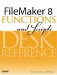# NPV()

Category: Financial

 Syntax: NPV ( payment; interestRate )

Parameters:

paymentA repeating field that contains one or more values representing loan and payment amounts.

interestRateAn interest rate, expressed as a decimal number.

Data type returned: Number

Description:

Returns the Net Present Value of a series of unequal payments made at regular intervals, assuming a fixed interestRate per interval. The repeating field specified in the first parameter should contain all loan and payment amounts.

Examples:

Imagine someone borrows \$300 from you and repays you \$100, \$50, \$100, and \$125 at regular intervals.

Assuming an interest rate of 5%, the NPV() function can tell you the actual profit, in todays dollars, that will be realized from this transaction. To calculate this, you would place the following values in a repeating number field: -300, 100, 50, 100, and 125. Then, use the formula

``` Round (NPV (Payments; .05)),
```

which returns \$28.39. Your actual profit on the transaction would be \$75 (simply the sum of the payments minus the original loan). That \$75, however, is collected over time, so the present value is discounted by the assumed interest rate. The higher the interest rate, the less the NPV of the \$75.FileMaker 8 Functions and Scripts Desk Reference
ISBN: 0789735113
EAN: 2147483647
Year: 2004
Pages: 352